## Q&A - How is loop gain important for op-amp gain accuracy - Apex

April 1, 2021

Q?

How is loop gain important for op-amp gain accuracy

A!

Loop gain is a measurement concept that can be useful when assessing the gain accuracy of an Op-Amp circuit across a range of frequencies. It refers to the difference in magnitude between the closed loop gain configuration of the circuit and the open loop gain characteristics of the Op-Amp and decreases with frequency as open loop gain decreases.

﻿This quantity can be measured by using the small signal response graph of an amplifier. The measurement process can be seen here through the Apex PA164 small signal response graph: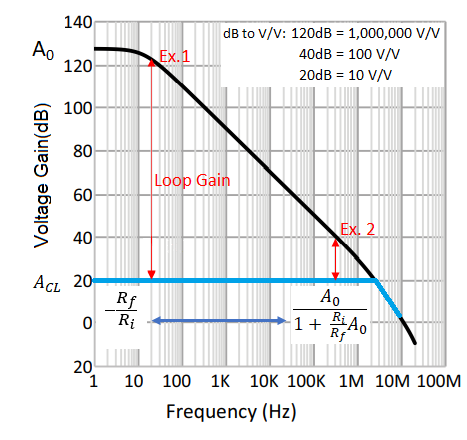One of the characteristics of an ideal Op-Amp is infinite open loop gain (A0). Along with this ideality comes the simplified approximation for inverting closed loop gain of a circuit based on the relationship between input and feedback resistors: ACL = -Rf/Ri.

In realistic situations, when open loop gain is not infinite, the approximation for closed loop gain becomes slightly more complex as loop gain decreases (A=V/V):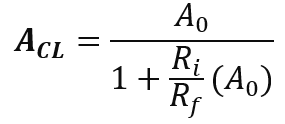Higher gain accuracy is achieved when there is more available loop gain (as seen on the left side of Figure 1) because the constant term ’1’ in the denominator of the complex closed loop equation becomes less significant.
Examples below illustrate this effect based on the simple circuit provided: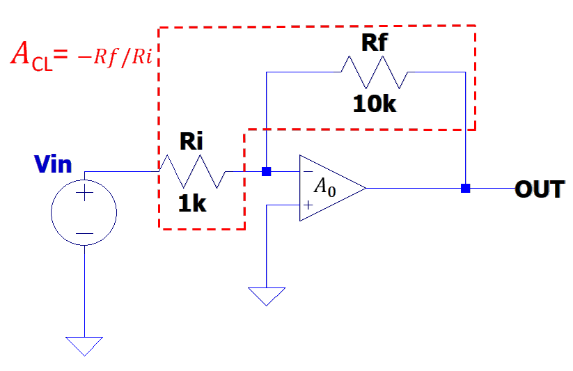Ex.1) A0=1,000,000 V/V, Ri=1k, Rf=10k, expected ACL=10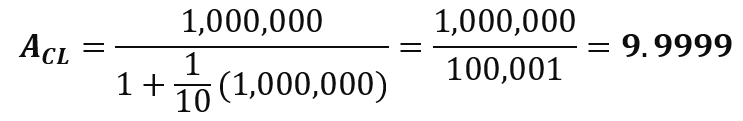With a loop gain of ~100dB there is no practical gain error or need to use the complex ACL equation.

﻿Ex.2) A0=100 V/V, Ri=1k, Rf=10k, expected ACL=10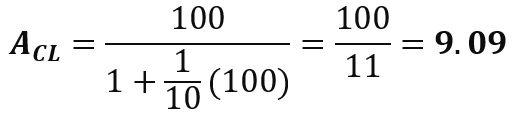With a loop gain of ~20dB there is a noticeable gain inaccuracy of roughly 9%.

When circuit conditions dictate that loop gain is going to be low, it is prudent to verify your gain accuracy and adjust your input conditions accordingly in order to achieve desired output amplitude.